Any equation that can be rearranged to the formcan be solved with the quadratic formula. The formula will lead to one of:

• two, real, distinct solutions
• one, real, repeated solution
• no real solutions

As we have seen on factored form to graph, the solutions toare the-intercepts of the parabola.

This parabola has two, real, distinct-intercepts: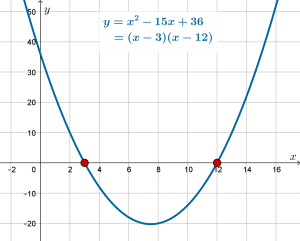This parabola has one, real, repeated-intercept: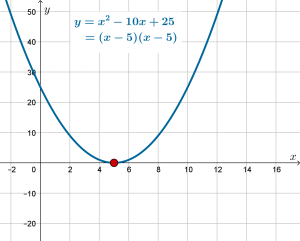This parabola has no real-intercepts. (We can define complex solutions but these are not included in this course).# Solution by factoring

If the solutions of the equationare whole numbers, or even reasonably simple fractions, the most efficient method to solve the equation is by factoring.

For example:To find the-intercepts we solve:If, however, the parabola cuts the-axis at points other than integer values it is not easy to factor. For example: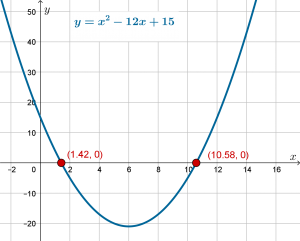The solutionsand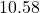are found using the quadratic formula.

Ifthen# How it works

SolveThis equation is already in the form, so it is easy to identify the parametersand.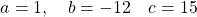Substitute these values to the formula we have:We can now type these two values (the plus and the minus) into the calculator:# Practice

Kuta Software worksheet to practice  applying the quadratic formula.

Find the roots of the parabolas in this applet. Use the quadratic formula, or perhaps factor if the expression factors easily. Round answers correct to 2 decimal places.